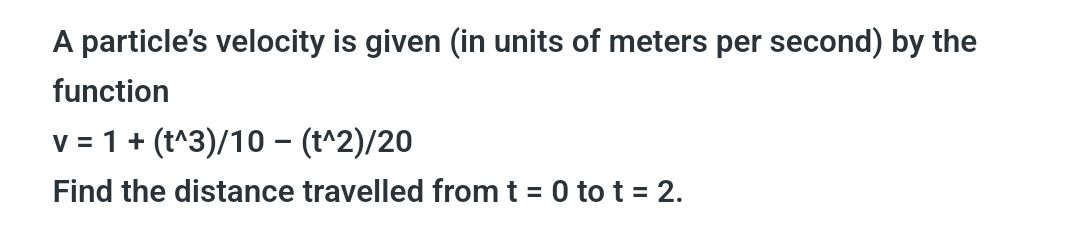### ¿Todavía tienes preguntas de matemáticas?

Pregunte a nuestros tutores expertos
Algebra
PreguntaA particle's velocity is given (in units of meters per second) by the function

$$v = 1 + ( t ^ { \wedge } 3 ) / 10 - ( t ^ { \wedge } 2 ) / 20$$

Find the distance travelled from $$t = 0$$ to $$t = 2$$ .